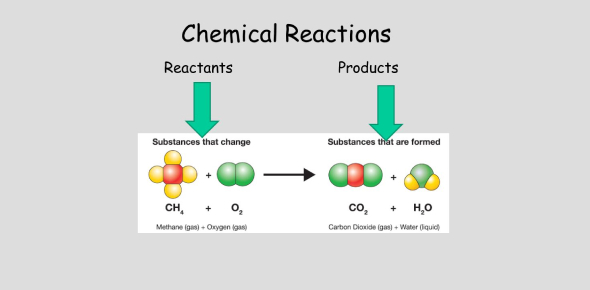# The Chemical Reaction - Quiz -

17 Questions | Attempts: 6114Settings.

• 1.
Pb(NO3)2 + 2 KI ---> PbI2 + 2 KNO3 What constitutes this reaction?
• A.

Decomposition

• B.

Combustion

• C.

Double displacement

• D.

Synthesis

• 2.
In a chemical reaction, a hydrocarbon compound reacts with the diatomic element oxygen. What type of reaction is this?
• A.

Single replacement

• B.

Combustion

• C.

Formation

• D.

Double replacement

• 3.
Is the following reaction a synthesis, decomposition, single replacement, double replacement, neutralization (acid-base), or combustion reaction? C10H8 + 12O2 → 10CO2 + 4H2O
• A.

Single replacement

• B.

Combustion

• C.

Synthesis

• D.

Decomposition

• 4.
Which of the following represents a chemically balanced decomposition reaction of nickel (III) oxide into its elements?
• A.

Ni2O3 + H2O2 → 2Ni + 3O2 + H2

• B.

2Ni3O2 → 4Ni + 3O2

• C.

2Ni2O3 → 4Ni + 3O2

• D.

2NiO → 2Ni + O2

• 5.
You can tell a chemical reaction because it always produces …
• A.

A different substance

• B.

Reactants

• C.

A change of state

• D.

Water

• 6.
Which one of the following is a chemical equation?
• A.

Ice + heat --> water

• B.

Iron + oxygen --> rust

• C.

CO2

• D.

C + O2 --> CO2

• 7.
BaCO3 --> BaO + CO2
• A.

Synthesis reaction

• B.

Decomposition reaction

• C.

Single replacement reaction

• D.

Double replacement reaction

• E.

Combustion reaction

• 8.
What is the product of neutralization?
• A.

Sugar

• B.

Salt

• C.

Water

• D.

Salt + water

• 9.
C2H5OH  +   3 O2   à   2 CO2 + 3H2O       Combustion
• A.

True

• B.

False

• 10.
9)      H2SO4    +      2NaOH   à       Na2SO +   2H2O    Decomposition
• A.

True

• B.

False

• 11.
Mg +      HCl  à    MgCl2  + H2   Single replacement
• A.

True

• B.

False

• 12.
Fe (II) + CuSO4 à FeSO4 + Cu  Single replacement
• A.

True

• B.

False

• 13.
Na2SO4 + BaCl2 à     2NaCl  + BaSO4     Double replacement
• A.

True

• B.

False

• 14.
2 Na(OH)     à    Na2O  +  H2O     Double replacement
• A.

True

• B.

False

• 15.
Is the following reaction a synthesis, decomposition, single replacement, double replacement, neutralization (acid-base), or combustion reaction? C10H8 + 12O2 → 10CO2 + 4H2O
• A.

Single replacement

• B.

Combustion

• C.

Synthesis

• D.

Decomposition

• 16.
Is the following reaction a synthesis, decomposition, single replacement, double replacement, neutralization (acid-base), or combustion reaction? C10H8 + 12O2 → 10CO2 + 4H2O
• A.

Single replacement

• B.

Combustion

• C.

Synthesis

• D.

Decomposition

• 17.
Is the following reaction a synthesis, decomposition, single replacement, double replacement, neutralization (acid-base), or combustion reaction? C10H8 + 12O2 → 10CO2 + 4H2O
• A.

Single replacement

• B.

Combustion

• C.

Synthesis

• D.

Decomposition

## Related TopicsBack to top
×

Wait!
Here's an interesting quiz for you.